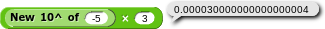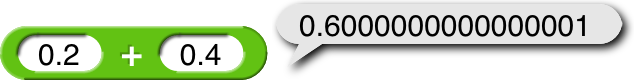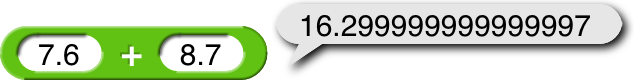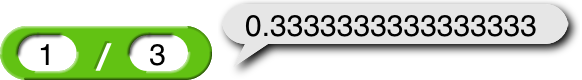# 10^ block is broken

The 10^ block is broken. It works fine when you are putting 10^ a positive number or zero. But the negative numbers have a lot of glitches. 10^-1 reports 0.1, as it should. 10^-2 and 10^-3 both are okay, but 10^-4 reports 0.00009999999999999999 exactly. I (and probably everyone else) know 10^-4 is 0.0001. 10^-5 reports 0.00000999999999999999. 10^-6 is okay. 10^-7 gives 1e-7, which I realize is okay, but it makes it hard to check if it actually is correct. Do you know what's going on?
loucheman made a block called New 10^ of, and it works really well, but when I multiply it by a number, it gives me that error again.As you can see, this calculation is very wrong. Is there a way to fix all of these errors?

Interestingly, 100^ is buggy too. Even weirder, the problem doesn't happen for 1000, 10000, 100000, and any other positive power of 10.

TL;DR: It's nothing to do with Snap!; it's a question of how computers represent non-integers, and will likely give exactly the same answer in any programming language.

Concise answer for mathematicians: There are uncountably many real numbers, even in a finite range, and only countably many possible bit patterns in a computer.

Long answer: Long strings of 9s are the tip of the iceberg:Note that that second example is off by three in the last decimal place, not just off by one. The underlying problem is that real numbers are dense, which means that between any two real numbers, no matter how close, there are infinitely many more numbers. Integers don't have this problem; there aren't any integers between 2 and 3.

What that means is that any pattern of bits inside the computer corresponds to infinitely many nearby numeric values. So, the bit pattern that represents 0.2 also represents values such as 0.199999999999999999999999999999999999999781 and 0.200000000000000000000000000000000004969. This doesn't bother you in a case likeeven though you know that an exact answer would require infinitely many threes.

So, in the case of 0.2+0.4, the problem isn't that computers can't represent 0.6 exactly (although they can't); it's that they can't represent 0.2 exactly and can't represent 0.4 exactly. And, as it happens, the exact value near 0.2 that is representable is just slightly more than 0.2. And same for 0.4. And when the computer adds these two numbers, each of which is slightly too big, the sum is enough too big to be visible.

Perhaps you think, "But 0.2 isn't like 1/3; it just has two digits!" That's true in base ten, but it's not true in base two, which is how numbers are represented inside the computer. The numbers that are representable exactly in a computer are powers of two and sums of finitely many powers of two. So 0.25 = 1/4 = 2^(-2) is exactly representable in base two. But 0.2 = 1/5 isn't.

The 52 bits of precision in 64-bit floating point format are equivalent to between 15 and 16 decimal digits, so if you round off the result values above to 15 digits you should get the right answer:

Perhaps Snap! should just always do that, round every displayed result (not every internal result) to 15 digits, so we won't get questions like this so often. :~)

Thanks! That explains everything really well!

I sense a new question: if the internal value does not match exactly with the displayed value, a person might be confused when [scratchblocks]<(expression) = (literal that matches displayed)>[/scratchblocks] returns false.

A little word play: you are very sensible. A patch solution creates a brand new challenge that requires it's own solution. What would be your solution to this new challenge?

Right, we'd have to round for comparisons too.